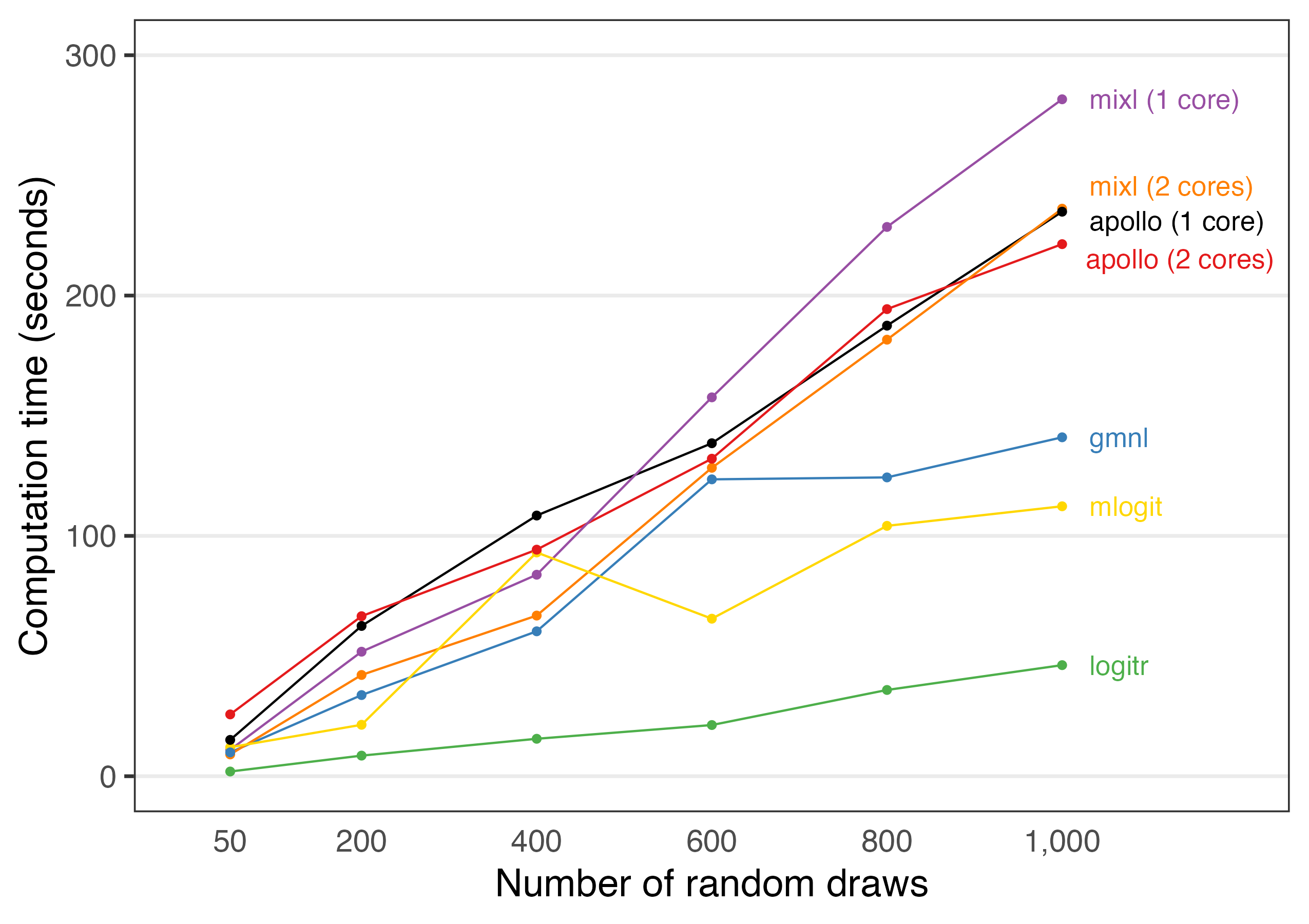# Benchmarking estimation speed against other packages

{logitr} is faster than most other packages with similar functionality. To demonstrate this, a benchmark was conducted by estimating the same preference space mixed logit model using the following R packages:

• {logitr}
• {mixl}
• {mlogit}
• {gmnl}
• {apollo}

The benchmark can be viewed at this Google Colab notebook:

Benchmarks will always vary for every run of a benchmarking code, even when run on the same machine due to variations in background processes. Thus, if you run this code yourself on a different machine, your results may vary, though the overall order and trends in terms of each package’s relative speed should be similar to those from the Colab notebook.

## Comparing run times

The {logitr} package includes a runtimes data frame that is exported from the Google Colab notebook used to conduct the benchmark. The tables below summarize the run times for each package and how many times slower they are relative to {logitr}.

library(logitr)
library(dplyr)
library(tidyr)
library(kableExtra) # For tables

numDraws <- unique(runtimes\$numDraws)
logitr_time <- runtimes %>%
filter(package == "logitr") %>%
rename(time_logitr = time_sec)
time_compare <- runtimes %>%
left_join(select(logitr_time, -package), by = "numDraws") %>%
mutate(mult = round(time_sec/ time_logitr, 1)) %>%
select(-time_logitr)
# Compare raw times
time_compare %>%
select(-mult) %>%
pivot_wider(names_from = numDraws, values_from = time_sec) %>%
kbl()
package 50 200 400 600 800 1000
logitr 1.956981 8.568663 15.57897 21.29589 35.88949 46.21824
mixl (1 core) 10.985394 51.813358 83.84448 157.64578 228.54502 281.66884
mixl (2 cores) 9.070937 42.128199 66.84492 128.33416 181.64115 236.14920
mlogit 12.053144 21.399773 93.10552 65.54310 104.17485 112.31945
gmnl 9.920456 33.763408 60.29489 123.52575 124.33332 141.02002
apollo (1 core) 15.116417 62.525899 108.46728 138.54715 187.48298 234.89636
apollo (2 cores) 25.722478 66.584539 94.27059 132.11713 194.35550 221.39257
# Compare how many times slower compared to logitr
time_compare %>%
select(-time_sec) %>%
pivot_wider(names_from = numDraws, values_from = mult) %>%
kbl()
package 50 200 400 600 800 1000
logitr 1.0 1.0 1.0 1.0 1.0 1.0
mixl (1 core) 5.6 6.0 5.4 7.4 6.4 6.1
mixl (2 cores) 4.6 4.9 4.3 6.0 5.1 5.1
mlogit 6.2 2.5 6.0 3.1 2.9 2.4
gmnl 5.1 3.9 3.9 5.8 3.5 3.1
apollo (1 core) 7.7 7.3 7.0 6.5 5.2 5.1
apollo (2 cores) 13.1 7.8 6.1 6.2 5.4 4.8

The code below plots the relative run times from the Colab notebook.

library(ggplot2)
library(ggrepel)

plotColors <- c("black", RColorBrewer::brewer.pal(n = 5, name = "Set1"), "gold")
benchmark <- runtimes %>%
ggplot(aes(x = numDraws, y = time_sec, color = package)) +
geom_line() +
geom_point() +
geom_text_repel(
data = . %>% filter(numDraws == max(numDraws)),
aes(label = package),
hjust = 0, nudge_x = 40, direction = "y",
size = 4.5, segment.size = 0
) +
scale_x_continuous(
limits = c(0, 1200),
breaks = numDraws,
labels = scales::comma) +
scale_y_continuous(limits = c(0, 300), breaks = seq(0, 300, 100)) +
scale_color_manual(values = plotColors) +
guides(
point = guide_legend(override.aes = list(label = "")),
color = guide_legend(override.aes = list(label = ""))) +
theme_bw(base_size = 18) +
theme(
panel.grid.minor = element_blank(),
panel.grid.major.x = element_blank(),
legend.position = "none",
axis.line.x = element_blank(),
axis.ticks.x = element_blank()
) +
labs(
x = "Number of random draws",
y = "Computation time (seconds)"
)

benchmark